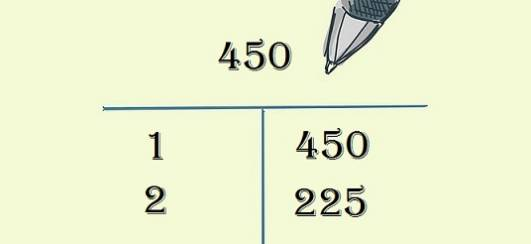# What Are All The Factors Of 450?

Since it ends in 0, you can tell right away 450 isn't a prime number, and therefore has more than two factors. There's no easy way to work those factors out, so you have to do some maths and make some notes. And it turns out that 450 has 18 factors, or 36 including negatives.

Start off by remembering that 450 is a factor of 450, by multiplying it by 1. That means 450 and 1 are both factors.

Then you try the next number - 2 multiplied by 225 equals 450, and so 2 and 225 are also both factors.Keep going up until you come to the middle point. In this case, it's 18 and 25. After 18, the next factor of 450 is 25, which means there are no more factors, as you've already discovered them!

By this point, you should have found the following are all factors of 450:

1, 2, 3, 5, 6, 9, 10, 15, 18, 25, 30, 45, 50, 75, 90, 150, 225, and 450.

And don't forget that when you multiply two negative numbers together, you get a positive number. So the negatives of all the above are also factors: -1, -2, -3, and so on.

This tutorial should help give some visual cues to get the mathematical side of your brain going!